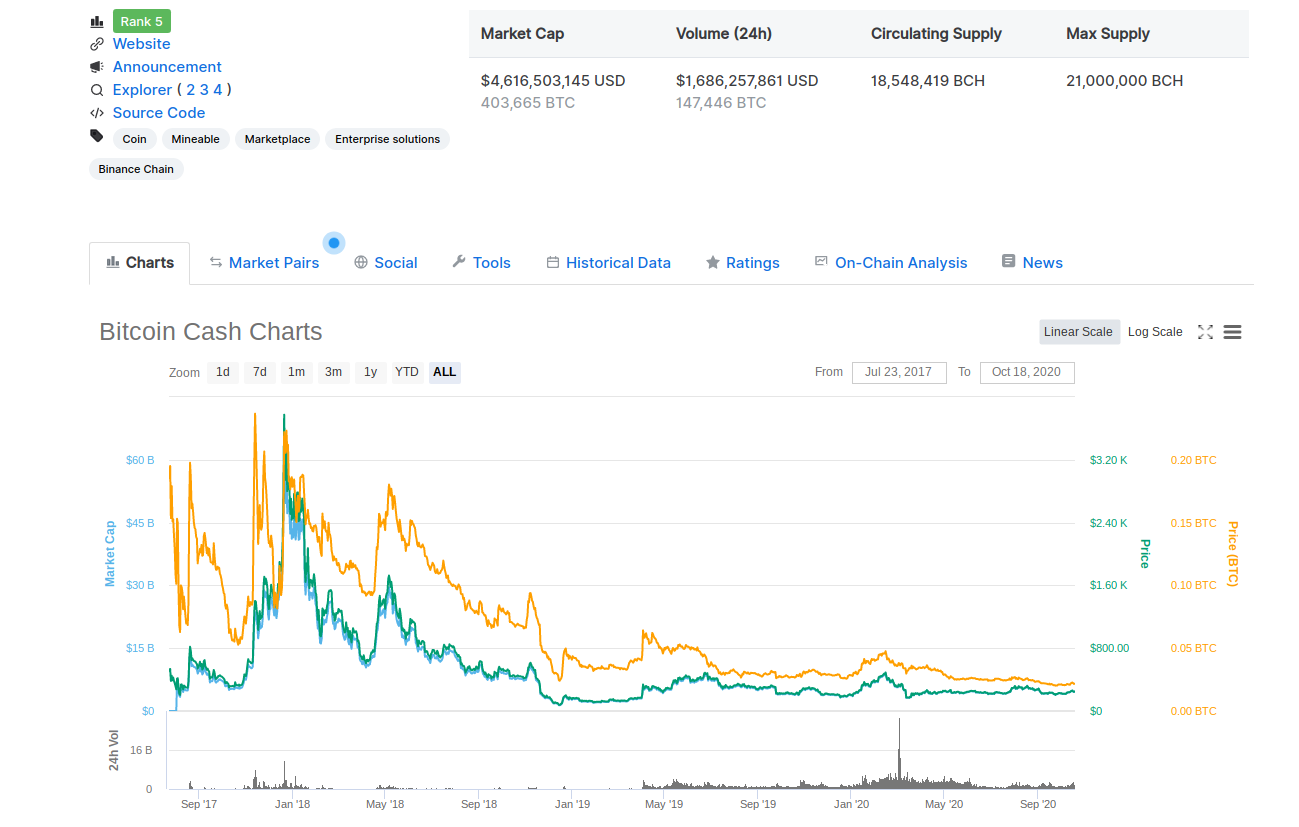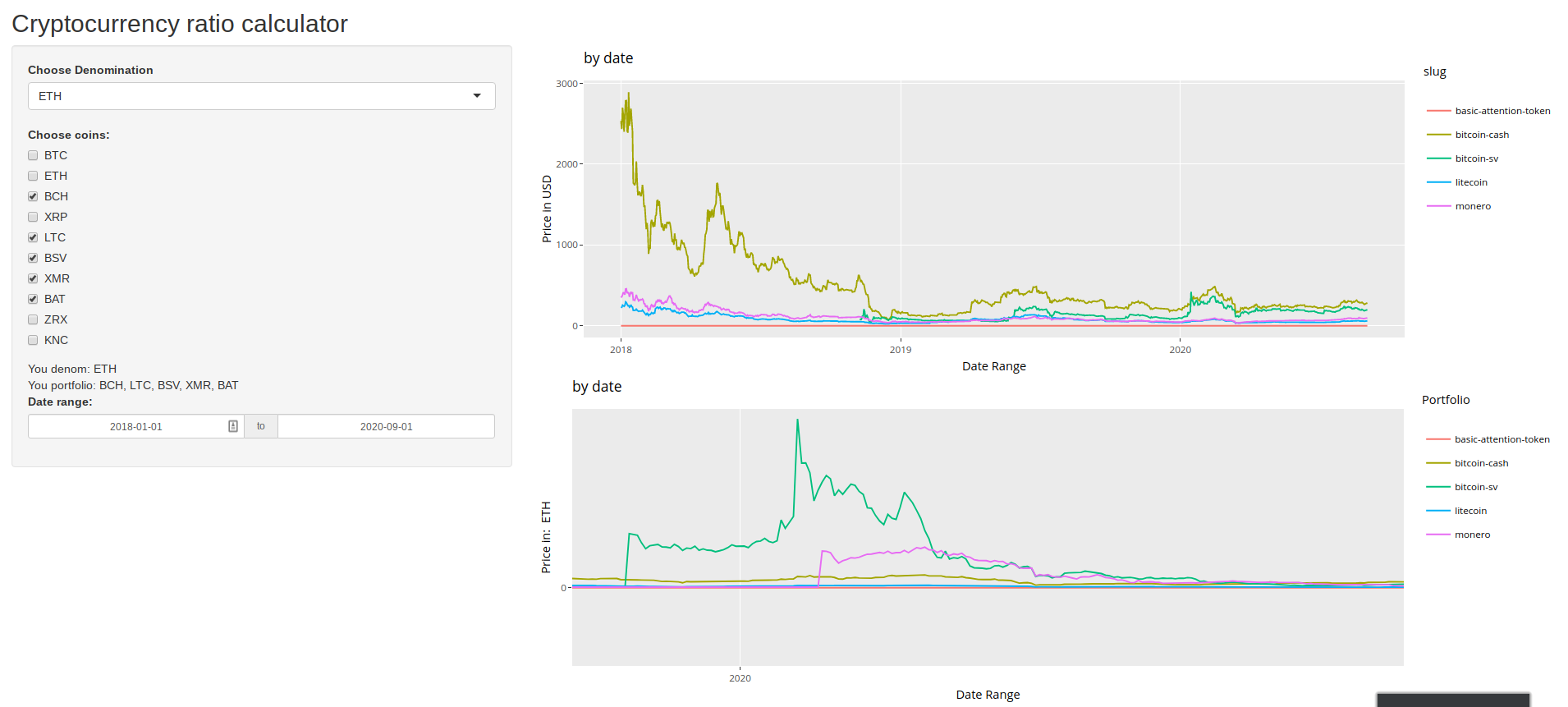# ShinyCoin - A Cryptocurrency Denomination Calculator in R

We all want to make more money but with so many currencies available someone can be “making money” in one currency while “losing money” if denominated in another. The global economy is confusing and adding hundreds of new “alternative digital currencies” into the mix has not helped.

In trading, many times I have wanted to see something like, how well my Ethereum (ETH) are doing in terms of Monero (XMR), or how many XRM can I get for my Bitcoin (BTC)? There are tons of tools like CoinMarketCap that let us see each coin denominated in USD, BTC and sometimes ETH, but I have never found a good tool that let me chose any denomination I want and to see multiple coins at once.The best we could do, until now!

So I’m building Shiny Coin, a price visualization tool written in R and using the Shiny package. It is still early stages but the pieces are in place and the goal is to to have a tool that can do the following:

• Load and save a user’s “portfolio” and coin to “denominate” in.
• Build interactive plots of the portfolio in USD and user denominated coin.
• Calculate ratios and identify regions of high deviance.
• Predict future price/ratio regression.ShinyCoin 0.1

The whole project is open source here on GitHub and the ShinyCoin app is running hosted by Shiny here. I am working to improve the interface and the prediction part I am not yet making public but would love any thoughts or feedback. Next I’ll walk through the R code to produce the above.

# First, lots of libraries

Crypto() is the most important package that lets us download daily price data for the top 100 coins. Makes the data loading step very easy. Plotly() gives us our interactive graphs and lubridate() obviously is used to lubricate our dates.

`library(shiny)library(crypto)library(remotes)library(crypto)library(ggplot2)library(lubridate)library(plotly)`

# Loading and cleaning the data

We start with just a list of coins that we want to use, must be all uppercase. Then to get the data we use the crypto_history() function from crypto() using that list of coins. In my case I am loading old data from a csv and just downloading the newest data each time to help increase the loading speed. Then use rbind() to stick the two together. Really, all you need is crypto_history() though.

`fullCoinList <- c("BTC", "ETH", "BCH", "XRP", "LTC", "BSV", "XMR", "BAT", "ZRX", "KNC")allCoinDataBeforeSep01 <- read.csv('allDataToSep1.csv', stringsAsFactors = FALSE)allCoinDataAfterSep01 <- crypto_history(fullCoinList, start_date = '20200901')allCoinDataBeforeSep01 <- dplyr::select(allCoinDataBefSep01, -1)allCoinDataBeforeSep01\$date <- as.Date(allCoinDataBeforeSep01\$date)allCoinDataAfterSep01\$date <- as.Date(allCoinDataAfterSep01\$date)allCoinData <- rbind(allCoinDataBeforeSep01, allCoinDataAfterSep01)allCoinData\$date <- as.Date(allCoinData\$date)`

# Get user input

In the “ui” function part of this app we are getting the user’s input of coins for the “portfolio”, the choice of the coin to denominate in and the date range. I am not crazy about the date input and the formatting was a huge pain but it works so am leaving it alone. This is where you would add more coins to choose from.

`ui <- fluidPage(  titlePanel("Cryptocurrency ratio calculator"),    sidebarLayout(      sidebarPanel(        selectizeInput(inputId = "coinDenom",                      label = "Choose Denomination",                      choices = unique(fullCoinList)),              checkboxGroupInput("coinCheckChoice", "Choose coins:",                         choiceNames =                           list("BTC", "ETH", "BCH", "XRP", "LTC", "BSV", "XMR", "BAT", "ZRX", "KNC"),                         choiceValues =                           list("BTC", "ETH", "BCH", "XRP", "LTC", "BSV", "XMR", "BAT", "ZRX", "KNC"),                         selected='BCH'),            textOutput('coinDenom'),      textOutput("workingCoinList"),      textOutput("coinCheckChoice"),      textOutput("dateRangeStart"),      textOutput("dateRangeEnd"),      dateRangeInput("dateRangeInput", "Date range:",                     start = "2018-01-01",                     end   = "2020-09-01"),),        mainPanel(      plotlyOutput("distPlot"),      plotlyOutput("distPlotRatio"),)))`

# Server function part 1

Here we just show the user what they just inputed with these two output statements.

`server <- function(input, output) {  output\$workingCoinList <- renderText({    coinList <- paste(input\$coinCheckChoice, collapse = ", ")    paste("You portfolio: ", coinList)  })    output\$coinDenom <- renderText({    coinDenom <- paste(input\$coinDenom)    paste("You denom: ", coinDenom)  })    output\$dateRangeInput <- renderText({    dateRangeInput <- paste(input\$dateRangeInput)    paste("You date: ", dateRangeInput)  })`

# Server function part 2

Output the ggplots. Plotly works just by wrapping the ggplot object which is very nice. I choose to filter the date here but this could probably be done better in a separate statement above.

`output\$distPlot <- renderPlotly({  denomPrice <- allCoinData %>% dplyr::filter(., symbol == input\$coinDenom) %>% dplyr::select(., open)  activeCoinData <- allCoinData %>% dplyr::mutate(., newOpen=(open/denomPrice\$open))   userDateInput <- as.Date(input\$dateRangeInput)   activeCoinData <- activeCoinData %>% dplyr::filter(., date >= as.Date(userDateInput) & date <= as.Date(userDateInput))  makeGraph(input\$coinCheckChoice, userDateInput, userDateInput, activeCoinData)  })output\$distPlotRatio <- renderPlotly({    denomPrice <- allCoinData %>% dplyr::filter(., symbol == input\$coinDenom) %>% dplyr::select(., open)    activeCoinData <- allCoinData %>% dplyr::mutate(., newOpen=(open/denomPrice\$open))    userDateInput <- as.Date(input\$dateRangeInput)     activeCoinData <- activeCoinData %>% dplyr::filter(., date >= as.Date(userDateInput) & date <= as.Date(userDateInput))    makeGraphRatio(input\$coinCheckChoice, userDateInput, userDateInput, activeCoinData, input\$coinDenom)  })}shinyApp(ui = ui, server = server)`

# Finally, the makeGraph() function

makeGraph just ouputs the ggplot object and gives titles and labels to our two plots.

`makeGraph <- function(coinList, startDate, endDate, df){  df <- df %>% dplyr::filter(., symbol %in% coinList )  graph <- ggplotly(ggplot(df, aes(x = date, y = open, col=slug))+    geom_line() +  labs(title = "Price in BTC", y= "Price in USD", x = "Date Range") +    ggtitle("Price in BTC"))  return(graph)}makeGraphRatio <- function(coinList, startDate, endDate, df, coinDenom){  df <- df %>% dplyr::filter(., symbol %in% coinList )  graph <- ggplotly(ggplot(df, aes(x = date, y = newOpen, col=slug))+    geom_line() + labs(colour = "Portfolio", title = paste("Price in ", coinDenom), y= paste("Price in: ", coinDenom) , x = "Date Range") +    ggtitle("by date"))  return(graph)}`

And that’s it. I am hoping to add an alert for when a ratio is out of its normal range as the whole point of this tool is to help me make money trading crypotcurrencies after all ;) Thanks for any thought and please reach out on Twitter too.

## Here is the full code in once block:

`library(shiny)library(crypto)library(remotes)library(crypto)library(ggplot2)library(lubridate)library(plotly)makeGraph <- function(coinList, startDate, endDate, df){  df <- df %>% dplyr::filter(., symbol %in% coinList )  graph <- ggplotly(ggplot(df, aes(x = date, y = open, col=slug))+    geom_line() +  labs(title = "Price in BTC", y= "Price in USD", x = "Date Range") +    ggtitle("Price in BTC"))  return(graph)}makeGraphRatio <- function(coinList, startDate, endDate, df, coinDenom){  df <- df %>% dplyr::filter(., symbol %in% coinList )  graph <- ggplotly(ggplot(df, aes(x = date, y = newOpen, col=slug))+    geom_line() + labs(colour = "Portfolio", title = paste("Price in ", coinDenom), y= paste("Price in: ", coinDenom) , x = "Date Range") +    ggtitle("by date"))  return(graph)}fullCoinList <- c("BTC", "ETH", "BCH", "XRP", "LTC", "BSV", "XMR", "BAT", "ZRX", "KNC")allCoinDataBeforeSep01 <- read.csv('allDataToSep1.csv', stringsAsFactors = FALSE)allCoinDataAfterSep01 <- crypto_history(fullCoinList, start_date = '20200901')allCoinDataBeforeSep01 <- dplyr::select(allCoinDataBeforeSep01, -1)allCoinDataBeforeSep01\$date <- as.Date(allCoinDataBeforeSep01\$date)allCoinDataAfterSep01\$date <- as.Date(allCoinDataAfterSep01\$date)allCoinData <- rbind(allCoinDataBeforeSep01, allCoinDataAfterSep01)allCoinData\$date <- as.Date(allCoinData\$date)ui <- fluidPage(  titlePanel("Cryptocurrency ratio calculator"),    sidebarLayout(      sidebarPanel(        selectizeInput(inputId = "coinDenom",                      label = "Choose Denomination",                      choices = unique(fullCoinList)),              checkboxGroupInput("coinCheckChoice", "Choose coins:",                         choiceNames =                           list("BTC", "ETH", "BCH", "XRP", "LTC", "BSV", "XMR", "BAT", "ZRX", "KNC"),                         choiceValues =                           list("BTC", "ETH", "BCH", "XRP", "LTC", "BSV", "XMR", "BAT", "ZRX", "KNC"),                         selected='BCH'),            textOutput('coinDenom'),      textOutput("workingCoinList"),      textOutput("coinCheckChoice"),      textOutput("dateRangeStart"),      textOutput("dateRangeEnd"),      dateRangeInput("dateRangeInput", "Date range:",                     start = "2018-01-01",                     end   = "2020-09-01"),),        mainPanel(      plotlyOutput("distPlot"),      plotlyOutput("distPlotRatio"),)))server <- function(input, output) {  output\$workingCoinList <- renderText({    coinList <- paste(input\$coinCheckChoice, collapse = ", ")    paste("You portfolio: ", coinList)  })    output\$coinDenom <- renderText({    coinDenom <- paste(input\$coinDenom)    paste("You denom: ", coinDenom)  })    output\$dateRangeInput <- renderText({    dateRangeInput <- paste(input\$dateRangeInput)    paste("You date: ", dateRangeInput)  })output\$distPlot <- renderPlotly({  denomPrice <- allCoinData %>% dplyr::filter(., symbol == input\$coinDenom) %>% dplyr::select(., open)  activeCoinData <- allCoinData %>% dplyr::mutate(., newOpen=(open/denomPrice\$open))   userDateInput <- as.Date(input\$dateRangeInput)   activeCoinData <- activeCoinData %>% dplyr::filter(., date >= as.Date(userDateInput) & date <= as.Date(userDateInput))  makeGraph(input\$coinCheckChoice, userDateInput, userDateInput, activeCoinData)  })output\$distPlotRatio <- renderPlotly({    denomPrice <- allCoinData %>% dplyr::filter(., symbol == input\$coinDenom) %>% dplyr::select(., open)    activeCoinData <- allCoinData %>% dplyr::mutate(., newOpen=(open/denomPrice\$open))    userDateInput <- as.Date(input\$dateRangeInput)     activeCoinData <- activeCoinData %>% dplyr::filter(., date >= as.Date(userDateInput) & date <= as.Date(userDateInput))    makeGraphRatio(input\$coinCheckChoice, userDateInput, userDateInput, activeCoinData, input\$coinDenom)  })}shinyApp(ui = ui, server = server)`

## Analytics Vidhya

Analytics Vidhya is a community of Analytics and Data…

## Sign up for Analytics Vidhya News Bytes

### By Analytics Vidhya

Latest news from Analytics Vidhya on our Hackathons and some of our best articles! Take a look.

By signing up, you will create a Medium account if you don’t already have one. Review our Privacy Policy for more information about our privacy practices.

Check your inbox
Medium sent you an email at to complete your subscription.

## Analytics Vidhya

Analytics Vidhya is a community of Analytics and Data Science professionals. We are building the next-gen data science ecosystem https://www.analyticsvidhya.com

Written by

## Kyle Benzle

I am a plant biologist with an MS from OSU and broad experience in data science, cell biology, genetics, genomics, and plant breeding.## Analytics Vidhya

Analytics Vidhya is a community of Analytics and Data Science professionals. We are building the next-gen data science ecosystem https://www.analyticsvidhya.com

## More From Medium

Medium is an open platform where 170 million readers come to find insightful and dynamic thinking. Here, expert and undiscovered voices alike dive into the heart of any topic and bring new ideas to the surface. Learn more

Follow the writers, publications, and topics that matter to you, and you’ll see them on your homepage and in your inbox. Explore

If you have a story to tell, knowledge to share, or a perspective to offer — welcome home. It’s easy and free to post your thinking on any topic. Write on Medium